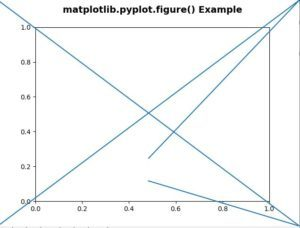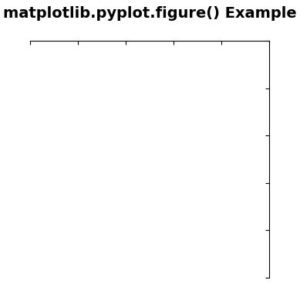Related Articles
matplotlib.pyplot.figure() in Python
• Difficulty Level : Basic
• Last Updated : 22 Apr, 2020

Matplotlib is a library in Python and it is numerical – mathematical extension for NumPy library. Pyplot is a state-based interface to a Matplotlib module which provides a MATLAB-like interface. There are various plots which can be used in Pyplot are Line Plot, Contour, Histogram, Scatter, 3D Plot, etc.

## matplotlib.pyplot.figure() Function:

The figure() function in pyplot module of matplotlib library is used to create a new figure.

Syntax: matplotlib.pyplot.figure(num=None, figsize=None, dpi=None, facecolor=None, edgecolor=None, frameon=True, FigureClass=, clear=False, **kwargs)

Parameters: This method accept the following parameters that are described below:

• num : This parameter is provided, and a figure with this id already exists.
• figsize(float, float): These parameter are the width, height in inches.
• dpi : This parameter is the resolution of the figure.
• facecolor : This parameter is the the background color.
• edgecolor : This parameter is the border color.
• frameon : This parameter suppress drawing the figure frame.
• FigureClass : This parameter use a custom Figure instance.
• clear : This parameter if True and the figure already exists, then it is cleared.

Return value: This method returns the following values:

• figure : This returns the Figure instance returned will also be passed to new_figure_manager in the backends.

Below examples illustrate the matplotlib.pyplot.figure() function in matplotlib.axes:

Example #1:

 `# Implementation of matplotlib function``import` `matplotlib.pyplot as plt``import` `matplotlib.lines as lines`` ` ` ` `fig ``=` `plt.figure()``fig.add_artist(lines.Line2D([``0``, ``1``, ``0.5``], [``0``, ``1``, ``0.3``]))``fig.add_artist(lines.Line2D([``0``, ``1``, ``0.5``], [``1``, ``0``, ``0.2``]))`` ` `plt.title(``'matplotlib.pyplot.figure() Example\n'``,``                ``fontsize ``=` `14``, fontweight ``=``'bold'``)`` ` `plt.show()`

Output:Example #2:

 `# Implementation of matplotlib function``import` `matplotlib.pyplot as plt``from` `mpl_toolkits.axisartist.axislines ``import` `Subplot`` ` `fig ``=` `plt.figure(figsize ``=``(``4``, ``4``))`` ` `ax ``=` `Subplot(fig, ``111``)``fig.add_subplot(ax)`` ` `ax.axis[``"left"``].set_visible(``False``)``ax.axis[``"bottom"``].set_visible(``False``)`` ` `plt.title(``'matplotlib.pyplot.figure() Example\n'``, ``                ``fontsize ``=` `14``, fontweight ``=``'bold'``)`` ` `plt.show()`

Output:Attention geek! Strengthen your foundations with the Python Programming Foundation Course and learn the basics.

To begin with, your interview preparations Enhance your Data Structures concepts with the Python DS Course. And to begin with your Machine Learning Journey, join the Machine Learning – Basic Level Course

My Personal Notes arrow_drop_up